# Volume - math word problems

1. Cheops pyramid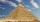The Pyramid of Cheops is a pyramid with a square base with a side of 233 m and a height of 146.6 m. It made from limestone with a density of 2.7 g/cm3. Calculate the amount of stone in tons. How many trains with 30 twenty tons wagons carry the stone?
2. Frustum of a cone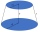A reservoir contains 28.54 m3 of water when completely full. The diameter of the upper base is 3.5 m while at the lower base is 2.5 m. Determine the height if the reservoir is in the form of a frustum of a right circular cone.
3. Alcohol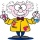6l 48% alcohol, how much 52% alcohol must be added to give 50% alcohol?
4. Cylinder and its circumferenceIf the height of a cylinder is 4 times its circumference c, what is the volume of the cylinder in terms of its circumference, c?
5. Body diagonalCalculate the volume of a cuboid whose body diagonal u is equal to 6.1 cm. Rectangular base has dimensions of 3.2 cm and 2.4 cm
6. Barrel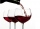Barrel of wine is filled by 9/14. What part of the barrel will remain filled when 1/4 of the wine we pour from the barrel?
7. Secret treasureScouts have a tent in the shape of a regular quadrilateral pyramid with a side of the base 4 m and a height of 3 m. Determine the radius r (and height h) of the container so that they can hide the largest possible treasure.
8. The cuboidThe cuboid is filled to the brim with water. The external dimensions are 95 cm, 120 cm, and 60 cm. The thickness of all walls and the bottom is 5 cm. How many liters of water fit into the cuboid?
9. Tetrahedral pyramidA regular tetrahedral pyramid is given. Base edge length a = 6.5 cm, side edge s = 7.5 cm. Calculate the volume and the area of its face (side area).
10. Alien shipThe alien ship has the shape of a sphere with a radius of r = 3000m, and its crew needs the ship to carry the collected research material in a cuboid box with a square base. Determine the length of the base and (and height h) so that the box has the large
11. The cylinder 2Find the volume and the lateral area of a cylinder of height 12 inches and a base radius of 4 inches.
12. Space diagonalThe space diagonal of a cube is 129.91 mm. Find the lateral area, surface area and the volume of the cube.
13. Three glasses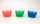Three glasses of different colors have different volumes. Red 1.5 liter is filled from 2/5, blue 3/4 liter is filled from 1/3, and the third green 1.2 liter is empty. Pour green glass 1/4 of the contents from the red glass and 2/5 of the content from the
14. Water in vegetables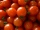Tomatoes in the store contain 99% water. After being transported to the shop, they were slightly dried and contained only 98% of water. How many kgs of tomatoes are in the store if there were 300 kg in stock?`
15. Wood prismsHow many weight 25 prisms with dimensions 8x8x200 cm? 1 cubic meter of wood weighs 800 kg.
16. Tank of fuelA 14.5-gallon tank of fuel is 3/4 full. How many more gallons will it take to fill up the tank?
17. Right pyramid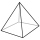A right pyramid on a base 4 cm square has a slant edge of 6 cm. Calculate the volume of the pyramid.
18. Right circular coneThe volume of a right circular cone is 5 liters. Calculate the volume of the two parts into which the cone is divided by a plane parallel to the base, one-third of the way down from the vertex to the base.
19. Rainwater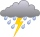The garden area of 800 square meters fell 3mm of rainwater. How many 10 liters of water can we water this garden equally?
20. Camel and water84% of the camel's weight is water. After drinking, its weight increased to 832 kg and water accounted for 85% of its weight. How much did it weigh before drinking?

Do you have an interesting mathematical word problem that you can't solve it? Submit math problem, and we can try to solve it.

We will send a solution to your e-mail address. Solved examples are also published here. Please enter the e-mail correctly and check whether you don't have a full mailbox.

Please do not submit problems from current active competitions such as Mathematical Olympiad, correspondence seminars etc...